﻿ IIT Gate Statistics Coaching Delhi, JAM 2018-19 Entrance, Best IIT Gate Coaching in Delhi, Best IIT Gate Statistics Coaching, IIT Gate Entrance 2018-19•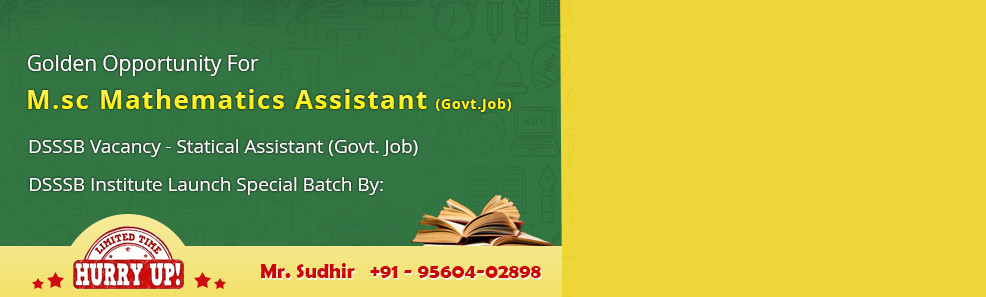•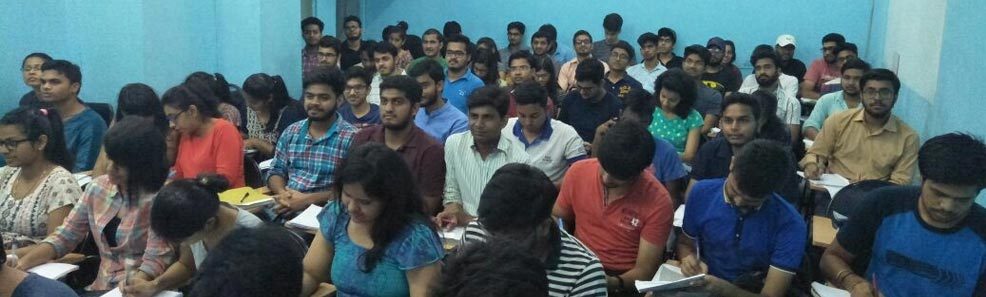•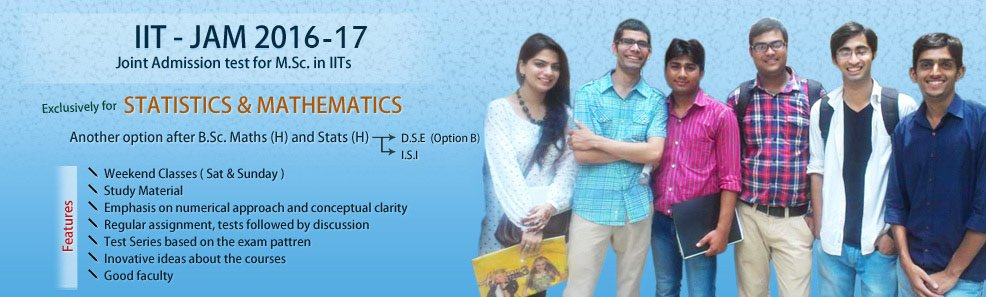•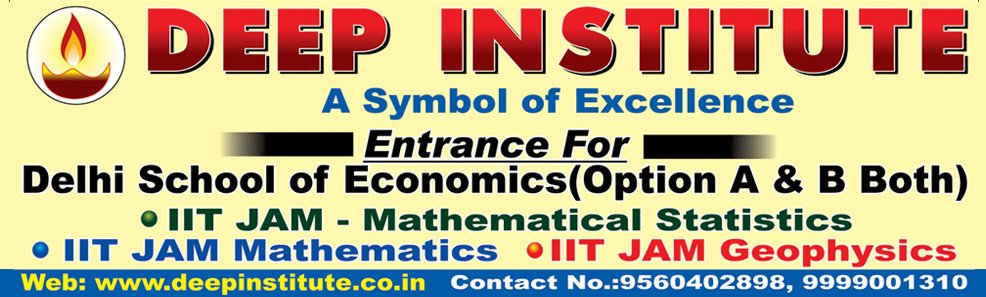•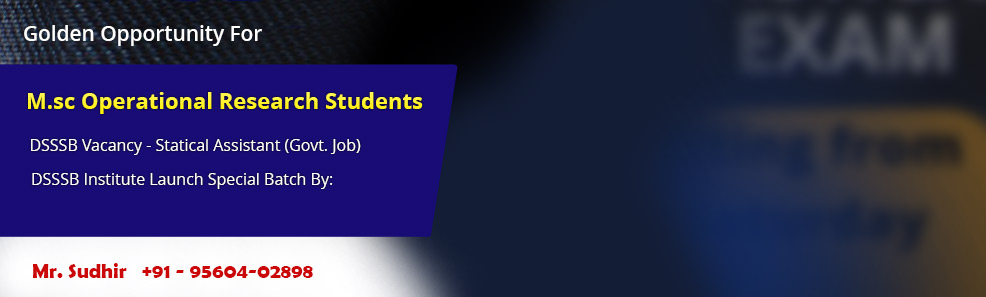•# IIT Gate Statistics

IIT Madras is going to conduct Graduate Aptitude Test in Engineering in Statistics subject from this year onwards. Gate is going to be conducted on 02, 03, 09 and 10 February, 2019 and in two sessions: Forenoon- 09:00 A.M. to 12:00 P.M. and Afternoon- 02:00 P.M. to 05:00 P.M. The Gate in Statistics is for those students who want to pursue Master and Doctoral Degree from IITs as well as various Public Sector Units consider Gate score of Candidates for recruitment. Calculus, Linear Algebra, Probability, Stochastic Processes, Inference, Regression Analysis, Multivariate Analysis, and Design of Experiments are important topics that will form the basis of the exam. The exam will be of 3 hours will various multiple choice questions as well as numerical answer type questions, making a total of around 65 questions. Deep Institute is the premier institute for providing best guidance and coaching for Gate Statistics by providing a student friendly environment of teaching and a good number of assignments on per class basis as well as an intensified test series for complete and best preparation. The detailed syllabus of Gate Statistics Exam is given below:

Calculus: Finite, countable and uncountable sets, Real number system as a complete orderedfield, Archimedean property; Sequences and series, convergence; Limits, continuity,uniform continuity, differentiability, mean value theorems; Riemann integration, Improperintegrals; Functions of two or three variables, continuity, directional derivatives, partialderivatives, total derivative, maxima and minima, saddle point, method of Lagrange'smultipliers; Double and Triple integrals and their applications; Line integrals and Surfaceintegrals, Green's theorem, Stokes' theorem, and Gauss divergence theorem.

Linear Algebra: Finite dimensional vector spaces over real or complex fields; Lineartransformations and their matrix representations, rank; systems of linear equations,eigenvalues and eigenvectors, minimal polynomial, Cayley-Hamilton Theorem,diagonalization, Jordan canonical form, symmetric, skew-symmetric, Hermitian, skew-Hermitian, orthogonal and unitary matrices; Finite dimensional inner product spaces, Gram- Schmidt orthonormalization process, definite forms.

Probability: Classical, relative frequency and axiomatic definitions of probability, conditional probability, Bayes' theorem, independent events; Random variables andprobability distributions, moments and moment generating functions, quantiles; Standarddiscrete and continuous univariate distributions; Probability inequalities (Chebyshev,Markov, Jensen); Function of a random variable; Jointly distributed random variables,marginal and conditional distributions, product moments, joint moment generating functions, independence of random variables; Transformations of random variables,sampling distributions, distribution of order statistics and range; Characteristic functions;Modes of convergence; Weak and strong laws of large numbers; Central limit theorem fori.i.d. random variables with existence of higher order moments.

Stochastic Processes: Markov chains with finite and countable state space, classification ofstates, limiting behaviour of n-step transition probabilities, stationary distribution, Poissonand birth-and-death processes.

Inference: Unbiasedness, consistency, sufficiency, completeness, uniformly minimumvariance unbiased estimation, method of moments and maximum likelihood estimations; Confidence intervals; Tests of hypotheses, most powerful and uniformly most powerfultests, likelihood ratio tests, large sample test, Sign test, Wilcoxon signed rank test, Mann- Whitney U test, test for independence and Chi-square test for goodness of fit.

Regression Analysis: Simple and multiple linear regression, polynomial regression,estimation, confidence intervals and testing for regression coefficients; Partial and multiplecorrelation coefficients.

Multivariate Analysis: Basic properties of multivariate normal distribution; Multinomialdistribution; Wishart distribution; Hotellings T2and related tests; Principal componentanalysis; Discriminant analysis; Clustering.

Design of Experiments: One and two-way ANOVA, CRD, RBD, LSD, 22and 23Factorialexperiments.